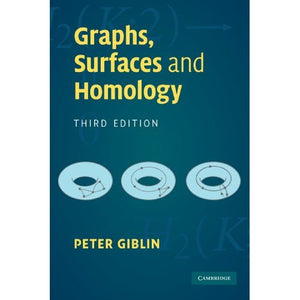># Graphs, Surfaces and Homology, Third Edition

• £11.09
• Save £18

Peter Giblin
Cambridge University Press
Edition: 3, 12/8/2010
EAN 9780521154055, ISBN10: 0521154057

Paperback, 272 pages, 22.8 x 15.2 x 1.6 cm
Language: English

Homology theory is a powerful algebraic tool that is at the centre of current research in topology and its applications. This accessible textbook will appeal to mathematics students interested in the application of algebra to geometrical problems, specifically the study of surfaces (sphere, torus, Mobius band, Klein bottle). In this introduction to simplicial homology - the most easily digested version of homology theory - the author studies interesting geometrical problems, such as the structure of two-dimensional surfaces and the embedding of graphs in surfaces, using the minimum of algebraic machinery and including a version of Lefschetz duality. Assuming very little mathematical knowledge, the book provides a complete account of the algebra needed (abelian groups and presentations), and the development of the material is always carefully explained with proofs given in full detail. Numerous examples and exercises are also included, making this an ideal text for undergraduate courses or for self-study.

Preface to the third edition
Preface to the first edition
List of notation
Introduction
1. Graphs
2. Closed surfaces
3. Simplicial complexes
4. Homology groups
5. The question of invariance
6. Some general theorems
7. Two more general theorems
8. Homology modulo 2
9. Graphs in surfaces
Appendix. Abelian groups
References
Index.